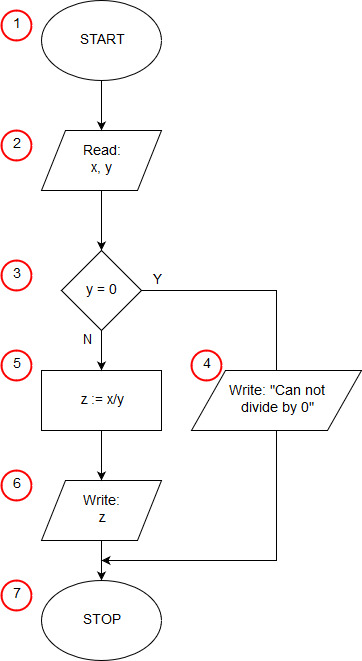# Algorithm of division

Apparently, the divide algorithm is trivial. The computer is able to perform basic mathematical operations, so it is enough to download the input data (valiant and divisor), perform the division operation and output the result, i.e. the quotient. And what if the divisor is equal to 0? We can not divide by 0, the program that will try to do so will end with an error. Our algorithm has to take this into account and check if it is feasible before attempting to divide numbers.Algorithm of division in: Pascal, C++, Java, Python, JavaScript

Description of the algorithm:

1. Start - our algorithm starts here.
2. We load input data - dividend x and y divisor.
3. We check if the divisor equals 0.
4. This way we go when the divisor is equal to 0, i.e. dividing is not feasible; we print a message saying that it can not be divided by 0.
5. The operating block calculating the quotient of the numbers x through y. In this place we can only find if y is different from 0.
6. After calculating the divide, we write the result saved in z.
7. Stop - the common end of all paths of the algorithm.